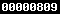GSE Algebra I GSE Geometry GSE Algebra II GSE PreCalc Other Courses Calculus I Adv. Mathematical Decision Making Analytical Geometry ```CP-Statistics ``` `All Units` Coordinate Algebra Integrated Algebra I Integrated Geometry GPS Middle School Math GIVE BACK TO MATT Home >Brain Teasers > Student ProjectsStudent Projects Short Video Project for Geometry 2000 Explaining the Fourth Dimension (Student Authors: Courtney Bassett, Michael Martinson, Dave Talamas) Chaos Gasket Program for Algebra 2 2001 - You can start with any number of placed vertices. Then, a point on the grid is selected at random. Next, one of the placed vertices is randomly selected and the midpoint is constructed between the 2 points. Then, from that new midpoint and another randomly selected placed vertex is selected and again, the midpoint between the 2 points is plotted. This process is repeated the number of desired times and interesting patterns form. (Student Author: Prashanth Reddy)